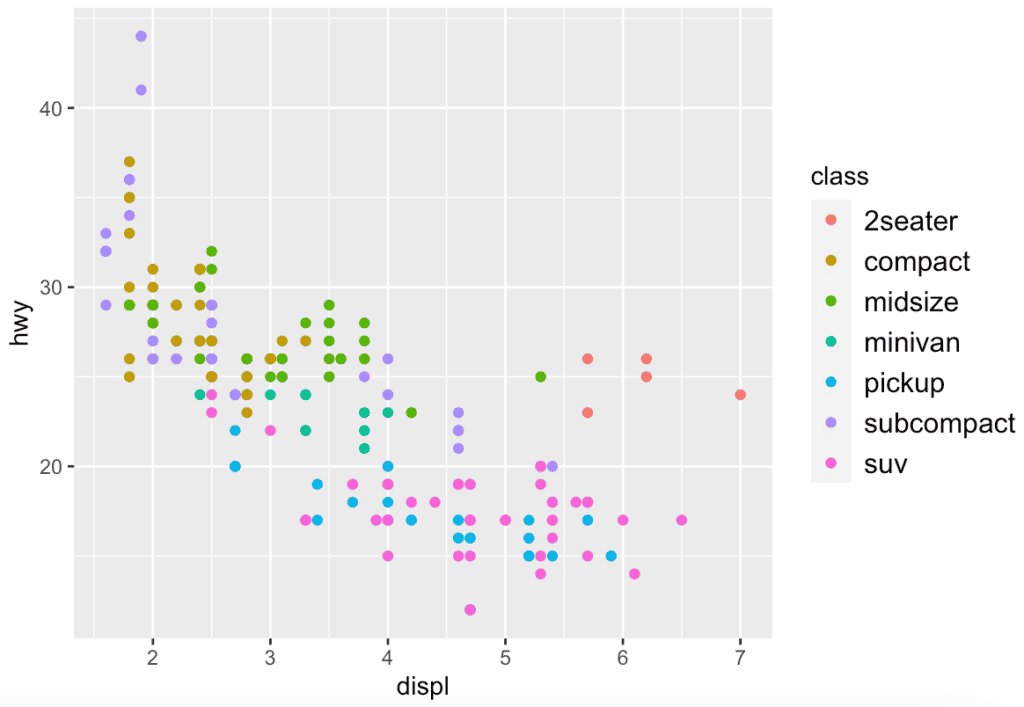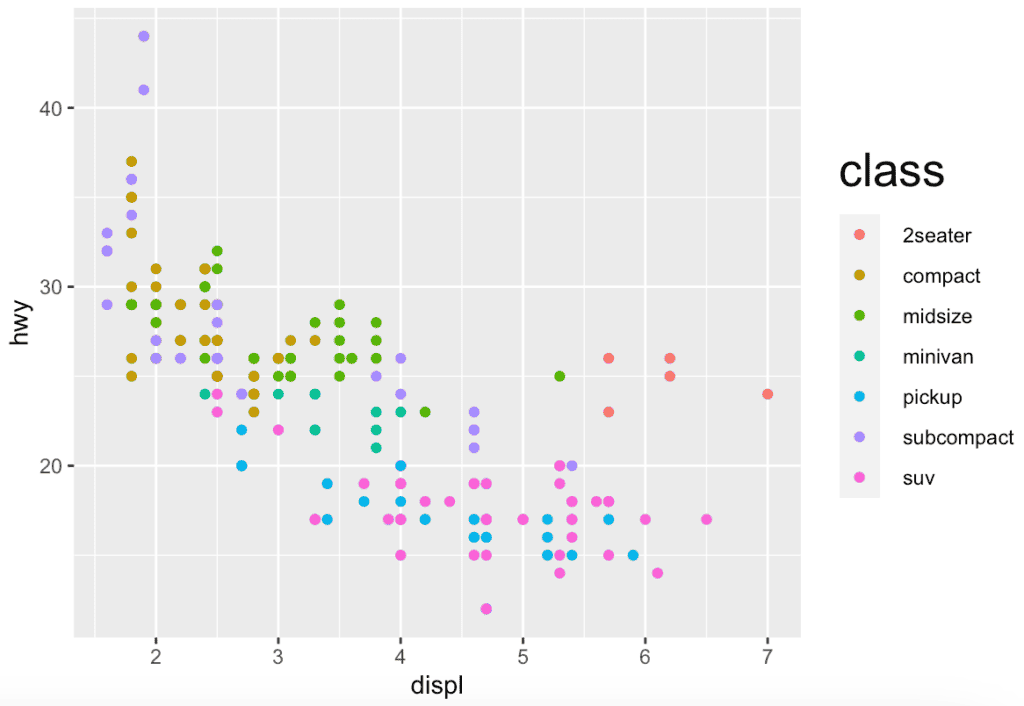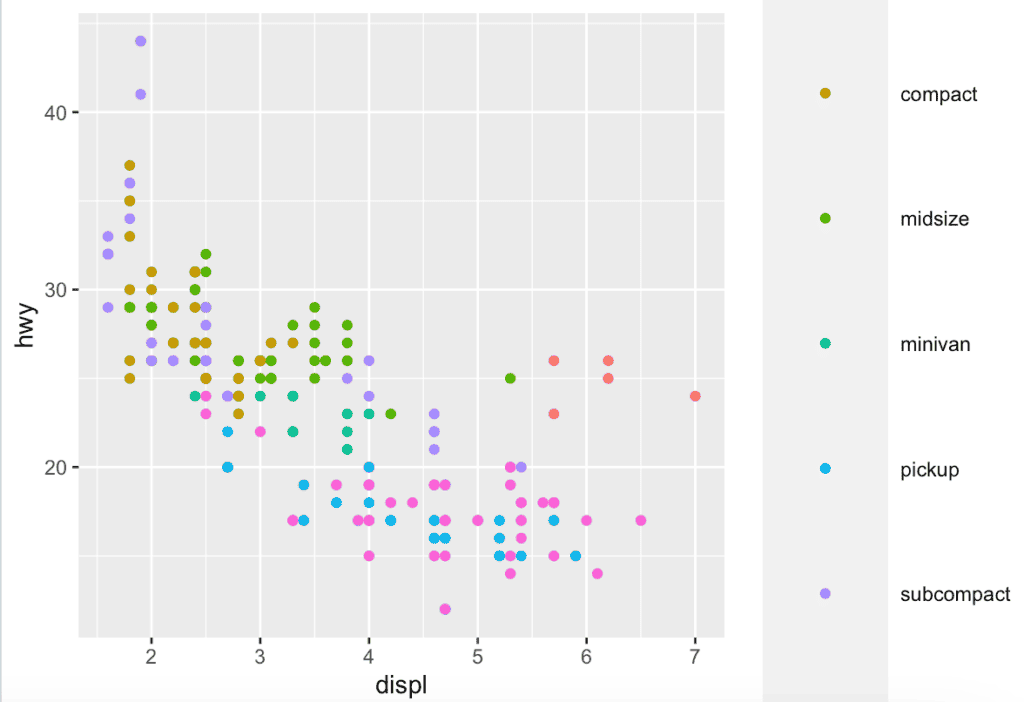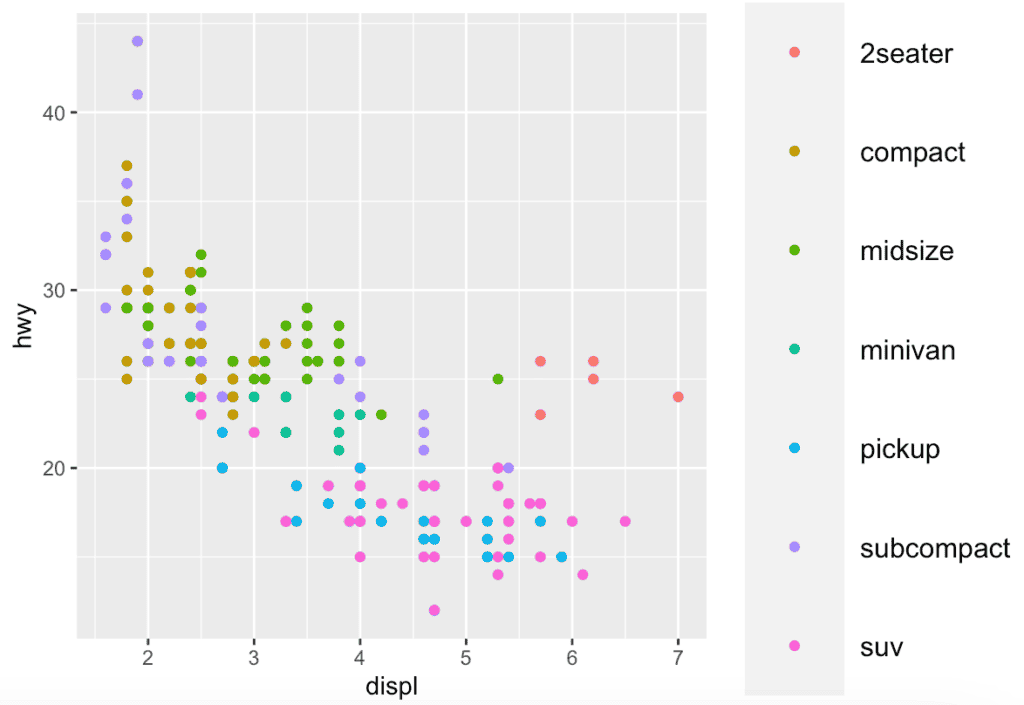# How to Change Legend Size in ggplot2 (With Examples)

To change the legend size of ggplot2 in R, you can use the “theme()” function, where you can control the text size, key size, and other aspects of the legend’s appearance.

## Syntax

``````ggplot(data, aes(x=x, y=y)) +
theme(legend.key.size = unit(1, 'cm'), #change legend key size
legend.key.height = unit(1, 'cm'), #change legend key height
legend.key.width = unit(1, 'cm'), #change legend key width
legend.title = element_text(size=14), #change legend title font size
legend.text = element_text(size=10)) #change legend text font size
``````

## Change Legend Text Size in R

You can change the size of the text in the legend using the legend.text argument inside the “theme()” function.

``````library(ggplot2)

# Create a scatter plot
p1 <- ggplot(mpg, aes(x = displ, y = hwy, color = class)) +
geom_point() +
theme(legend.text = element_text(size = 12))

# Display the plot
p1``````

Output## Change Legend Title Size in R

You can change the size of the legend title using the “legend.title” argument.

``````# Create a scatter plot with customized legend title size
p2 <- ggplot(mpg, aes(x = displ, y = hwy, color = class)) +
geom_point() +
theme(legend.title = element_text(size = 20))

# Display the plot
p2``````

Output## Change Legend Key Size

The legend key size (the size of the colored boxes, lines, or shapes) can be changed using the “legend.key.size” argument.

``````# Create a scatter plot with customized legend key size
p3 <- ggplot(mpg, aes(x = displ, y = hwy, color = class)) +
geom_point() +
theme(legend.key.size = unit(1.9, "cm"))

# Display the plot
p3``````

Output## Combine Multiple Changes

You can combine all these changes to customize the legend fully.

``````# Create a scatter plot with multiple customized legend settings
p4 <- ggplot(mpg, aes(x = displ, y = hwy, color = class)) +
geom_point() +
theme(
legend.text = element_text(size = 12), # Legend text size
legend.title = element_text(size = 14), # Legend title size
legend.key.size = unit(1.5, "cm") # Legend key size
)

# Display the plot
p4``````

OutputThat’s it!

## Related posts

How to Change the Legend Title in ggplot2

How to Remove a Legend in ggplot2

How to Change Legend Labels in ggplot2